Adding Mixed Numbers - Definition with Examples

The Complete K-5 Math Learning Program Built for Your Child

• 30 Million Kids

Loved by kids and parent worldwide

• 50,000 Schools

Trusted by teachers across schools

• Comprehensive Curriculum

Aligned to Common Core

A fraction consisting of a whole number as well as a fractional part is called a mixed number.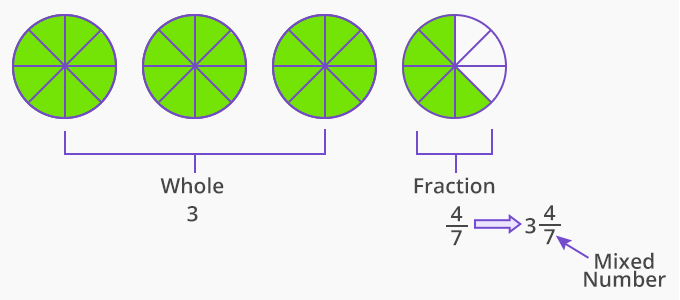Improper fractions, fractions greater than one, written in the form of a whole number and proper fraction are called mixed numbers.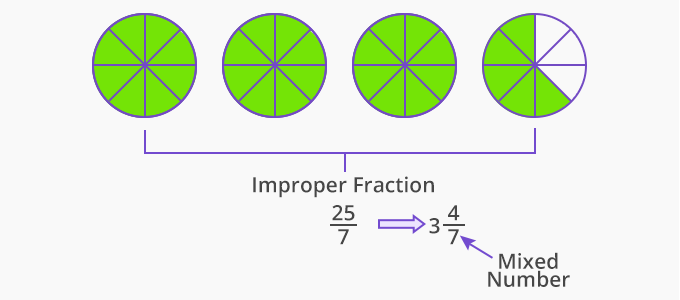Adding mixed numbers with like denominators

Let us see an example of the addition of mixed numbers with like denominators.

There are 2 4kg of apples in one basket and 3 35 kg of apples in another basket. How many apples are there altogether in both the baskets?

Here, to find the quantity of apples in both the baskets, we add the mixed numbers 2 4and 3 3. The denominators of both the fractional parts are the same. So, to add mixed numbers with like denominators we add the whole parts together and the fractional parts together and then combine the sum of the two as shown below: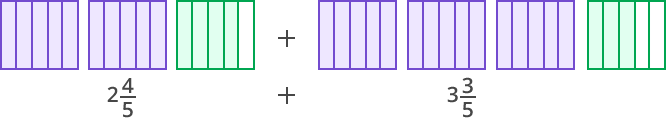Add the whole part with the whole part and the fractional part with the fractional part.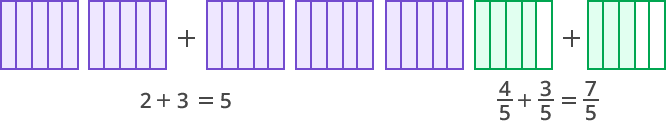If the sum of the fractional parts is an improper number, convert it to a mixed number.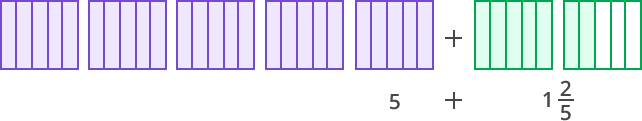Combine the sum of the wholes and fractions.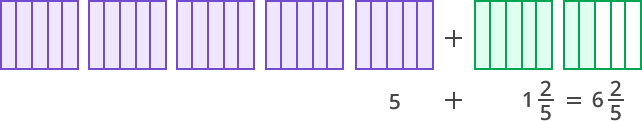Therefore, there are 6 25 kg of apples.

What we did:

45 + 3 35 = 2 + 3 + 45 + 35

= 5 + 75

= 5 + 1 25

= 6 25

Adding mixed numbers with unlike denominators

Now, let us look at an example to understand the addition of mixed numbers with unlike denominators.

Susan has 1 47 liters of orange juice. Keith has 2 25 liters of orange juice. How much orange juice do they have?

Here, to find the answer, we need to add mixed numbers 1 47 and 2 25 . Here, the denominators of both the fractional parts are different.

To add mixed numbers use any of the following methods:

Method 1:

• Add the whole numbers separately and add unlike fractions separately.
 Add   1 4⁄7  + 2 2⁄5 Adding whole numbers Adding Unlike fractions 1 + 2 = 3 4⁄7 + 2⁄5  LCM of 7 and 5 is 35.  Therefore  4⁄7 = 4⁄7  x  5⁄5 =  20⁄35       and 2⁄5  =  2⁄5  x  7⁄7 =  14⁄35  so, 4⁄7 + 2⁄5  =  20⁄35 +  14⁄35 = 34⁄35

• Combine the sum of the whole numbers and the fractional parts.

3 + 3435  = 3 3435

Method 2:

• Convert the mixed numbers to improper fractions.

1 47  = 117 and 2 25 = 125

• Add, unlike fractions.

1 47 + 2 25 = 117 + 125

Therefore,

LCM of 7 and 5 = 35

So,

117 = 117 x 55 = 5535

and

125 = 125 x 77 = 8435

Therefore,

117 + 125 = 5535 + 8435

= 13935

• Convert the improper fraction to a mixed number.

13935 = 3 3435

Won Numerous Awards & Honors